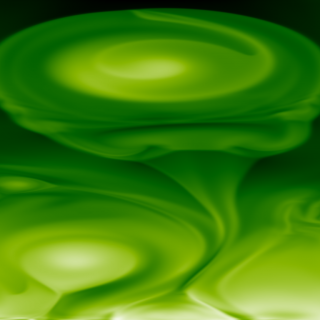# Self-similar Approach for Rotating Magnetohydrodynamic Solar and Astrophysical Structures

Luna, M.; Priest, E.; Moreno-Insertis, F.
Referencia bibliográfica

The Astrophysical Journal, Volume 863, Issue 2, article id. 147, 14 pp. (2018).

Fecha de publicación:
8
2018
Descripción
Rotating magnetic structures are common in astrophysics, from vortex tubes and tornadoes in the Sun all the way to jets in different astrophysical systems. The physics of these objects often combine inertial, magnetic, gas pressure, and gravitational terms. Also, they often show approximate symmetries that help simplify the otherwise rather intractable equations governing their morphology and evolution. Here we propose a general formulation of the equations assuming axisymmetry and a self-similar form for all variables: in spherical coordinates (r, θ, ϕ), the magnetic field and plasma velocity are taken to be of the form {\boldsymbol{B}}={\boldsymbol{f}}(θ )/{r}n and {\boldsymbol{v}}={\boldsymbol{g}}(θ )/{r}m, with corresponding expressions for the scalar variables like pressure and density. Solutions are obtained for potential, force-free, and non-force-free magnetic configurations. Potential field solutions can be found for all values of n. Nonpotential force-free solutions possess an azimuthal component B ϕ and exist only for n ≥ 2 the resulting structures are twisted and have closed field lines but are not collimated around the system axis. In the non-force-free case, including gas pressure, the magnetic field lines acquire an additional curvature to compensate for an outward pointing pressure gradient force. We have also considered a pure rotation situation with no gravity, in the zero-β limit: the solution has cylindrical geometry and twisted magnetic field lines. The latter solutions can be helpful in producing a collimated magnetic field structure; but they exist only when n < 0 and m < 0: for applications they must be matched to an external system at a finite distance from the origin.